# Assignment Help With Pore Volume Due to Initial Water

## Change in Pore Volume Due to Initial Water and Rock Expansion

The component describing the reduction in the hydrocarbon pore volume due to the expansion of initial (connate) water and the reservoir rock cannot be neglected for an under saturated oil reservoir. The water compressibility cw and rock compressibility cf are generally of the same order of magnitude as the compressibility of the oil. The effect of these two components, however, can be generally neglected for gas-cap-drive reservoir or when the reservoir pressure drops below the bubble-point pressure. The compressibility coefficient c which describes the changes in the volume (expansion) of the fluid or material with changing pressure is given by: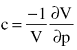Or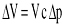Where Δ V represents the net changes or expansion of the material as a result of changes in the pressure. Therefore, the reduction in the pore volume due to the expansion of the connate water in the oil zone and the gas cap is given by:

Connate water expansion = [(pore volume) Swi] cw Δ p

Substituting for the pore volume (P.V)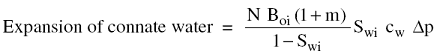Where

Δ p = change in reservoir pressure, (pi - p)

cw = water compressibility coefficient, psi−1

m = ratio of the volume of the gas-cap gas to the reservoir oil volume, bbl/b

Similarly, the reduction in the pore volume due to the expansion of the reservoir rock is given by: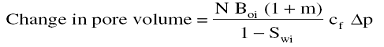Total changes in the pore volume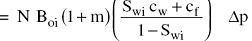### Pore Volume Occupied by the Injection Gas and Water

Assuming that Ginj volumes of gas and Winj volumes of water have been injected for pressure maintenance, the total pore volume occupied by the two injected fluids is given by:

Total volume = Ginj Bginj +Winj Bw

where

Ginj = cumulative gas injected, scf

Bginj = injected gas formation volume factor, bbl/scf

Winj = cumulative water injected, STB

Bw = water formation volume factor, bbl/STB

Combining all Equations and rearranging gives: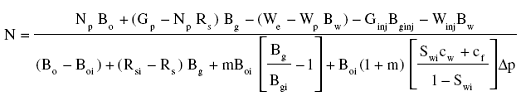Where

N = initial oil in place, STB

Gp = cumulative gas produced, scf

Np = cumulative oil produced, STB

Rsi = gas solubility at initial pressure, scf/STB

m = ratio of gas-cap gas volume to oil volume, bbl/bbl

Bgi = gas formation volume factor at pi, bbl/scf

Bginj = gas formation volume factor of the injected gas, bbl/scf

The cumulative gas produced Gp can be expressed in terms of the cumulative gas-oil ratio Rp and cumulative oil produced Np by:

Gp = Rp Np

Combining above two equations give: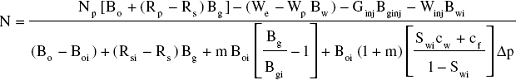The above relationship is referred to as the material balance equation (MBE). A more convenient form of the MBE can be determined by introducing the concept of the total (two-phase) formation volume factor Bt into the equation. This oil PVT property is defined as:

Bt = Bo + (Rsi - Rs) Bg

Introducing Bt into above equation and assuming, for sake of simplicity, no water or gas injection gives: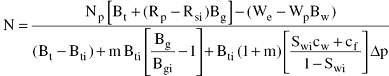Where

Swi = initial water saturation

Rp = cumulative produced gas-oil ratio, scf/STB

Δ p = change in the volumetric average reservoir pressure, psi.

In a combination drive reservoir where all the driving mechanisms are simultaneously present, it is of practical interest to determine the relative magnitude of each of the driving mechanisms and its contribution to the production: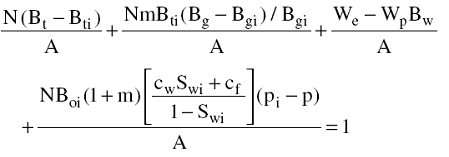With the parameter A as defined by:

A = Np [Bt + (Rp -Rsi) Bg]

Above equation can be abbreviated and expressed as:

DDI + SDI + WDI + EDI = 1.0

Where

DDI = depletion-drive index

SDI = segregation (gas-cap)-drive index

WDI = water-drive index

EDI = expansion (rock and liquid)-depletion index

The four terms of the left-hand side of above equation represent the major primary driving mechanisms by which oil may be recovered from oil reservoirs. As presented earlier in this chapter, these driving forces are:

## Depletion Drive

Depletion drive is the oil recovery mechanism Wherein the production of the oil from its reservoir rock is achieved by the expansion of the original oil volume with all its original dissolved gas. This driving mechanism is represented mathematically by the first term of main equation:

DDI = N (Bt - Bti)/A

Where

DDI is termed the depletion-drive index.

## Segregation Drive

Segregation drive (gas-cap drive) is the mechanism wherein the displacement of oil from the formation is accomplished by the expansion of the original free gas cap. This driving force is described by the second term of Equation:

SDI = [N m Bti (Bg - Bgi)/Bgi]/A

Where SDI is termed the segregation-drive index.

### Water Drive

Water drive is the mechanism wherein the displacement of the oil is accomplished by the net encroachment of water into the oil zone. This mechanism is represented by the third term of Equation:

WDI = (We -Wp Bw)/A

Where WDI is termed the water-drive index.

### Expansion Drive

For under saturated oil reservoirs with no water influx, the principle source of energy is a result of the rock and fluid expansion. Where all the other three driving mechanisms are contributing to the production of oil and gas from the reservoir, the contribution of the rock and fluid expansion to the oil recovery is too small and essentially negligible and can be ignored